Use the VBA StrConv function to convert ASCII strings to Upper, Lower or Proper case. The powerful StrConv function even has built in support for converting between strings, Unicode and ASCII Decimal character byte arrays.

This article will teach you the basics of the StrConv function, along with a few more advanced tricks.

## Introduction

### StrConv Arguments

The VBA StrConv function is available in Excel 2010 and greater. It returns a variant, typically a string, and accepts three arguments:

``StrConv(String, Conversion As VbStrConv, [LocaleID As Long])``
Argument Description
String The string that you want to convert. This can be a string you type directly into the formula, a variable or a cell.
Conversion How you want to convert the string.
LocaleID An optional argument that we’re going to ignore. By omitting the argument, the calculation uses your system’s Locale ID, which is usually what you’ll want to do.

Make powerful macros with our free VBA Developer Kit

This is actually pretty neat. If you have trouble understanding or remembering it, our free VBA Developer Kit can help. It’s loaded with VBA shortcuts to help you make your own macros like this one - we’ll send a copy, along with our Big Book of Excel VBA Macros, to your email address below.

I'll take a free VBA Developer Kit

### StrConv Conversion Types

You may be wondering what type of conversions the StrConv function is capable of performing. The following table summarizes the most useful conversions, which you would enter into the Conversions argument:

Value Conversion Description
1 vbUpperCase Converts string to uppercase. Equivalent to the VBA UCase function.
(Example: “EXCEL IS FUN.”)
2 vbLowerCase Converts string to lowercase. Equivalent to the VBA LCase function.
(Example: “excel is fun.”)
3 vbProperCase Converts string to proper case, where the first character of each word is capitalized.
(Example: “Excel Is Fun.”)
64 vbUnicode Converts string to its equivalent unicode value or byte array.
128 vbFromUnicode Converts string from its unicode value to an ANSI string or decimal byte array.

In your “Conversion” argument, you can either enter the Value or the Conversion entry from the table above. The following sections will walk you through a few examples.

## VBA Uppercase

This example converts a string to uppercase. Remember, you can also use this to convert the value stored in an Excel cell.

### vbUpperCase

``````Sub StrConvUpper()
Dim str1 As String
str1 = "Excel is fun."
MsgBox StrConv(str1, vbUpperCase)
End Sub``````

The string presented in the message box is identical to what would be created if you used the VBA UCase function.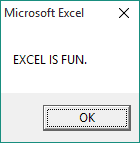vbUpperCase Results

## VBA Lowercase

This example converts a string to lowercase.

### vbLowerCase

``````Sub StrConvLower()
Dim str1 As String
str1 = "Excel is fun."
MsgBox StrConv(str1, vbLowerCase)
End Sub``````

As stated earlier, this is equivalent to using the VBA LCase function.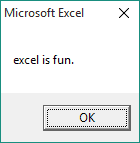vbLowerCase Results

## VBA Proper Case

This example converts a string to proper case, in which the first letter of each word is capitalized.

### vbProperCase

``````Sub StrConvProper()
Dim str1 As String
str1 = "Excel is fun."
MsgBox StrConv(str1, vbProperCase)
End Sub``````

Because it capitalizes the first letter of each word, proper case is ideal for beautifying a list of names or employees.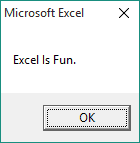vbProperCase Results

## VBA Unicode and Decimal

This is where things start to get interesting. You can use the vbUnicode conversion to store your string in a Unicode byte array, as shown in the next example:

### vbUnicode

``````Sub StrConvUnicode()
Dim str1 As String, str2 As String
Dim i As Integer
Dim x() As Byte

str1 = "Excel is fun."
x = StrConv(str1, vbUnicode)
For i = 0 To UBound(x)
str2 = str2 & " " & x(i)
Next i
MsgBox Trim(str2)
End Sub``````

Because we declared the variable x as a Byte array, each character in str1 is stored as a separate element in the array. The `For` loop isn’t an important part of the function. It just separates each element with a space so you can see what’s going on in the message box.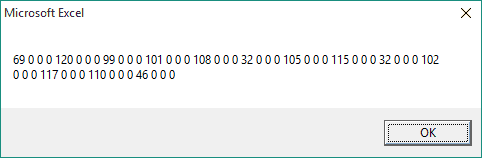vbUnicode Results

### vbFromUnicode

Likewise, we’re able to use the vbFromUnicode function to convert your string to a clean array of ANSI character codes, corresponding to the ASCII decimal values.

``````Sub StrConvFromUnicode()
Dim str1 As String, str2 As String
Dim i As Integer
Dim x() As Byte

str1 = "Excel is fun."
x = StrConv(str1, vbFromUnicode)
For i = 0 To UBound(x)
str2 = str2 & " " & x(i)
Next i
MsgBox Trim(str2)
End Sub``````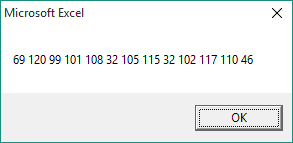vbFromUnicode Results

It’s not really necessary to use the StrConv function to convert to decimal arrays, but it’s still neat. The simpler way is to just set your byte array equal to your original string, without the StrConv function. However, if you want to use the above example, you can.

You could use the VBA chr command to return each decimal integer back to an ASCII character, but there’s an easier way. Keep reading to learn how.

## Converting Byte Arrays

Now that you know how to convert your strings to Unicode, you can take this one step further. The examples below show how to convert a Unicode byte string array or decimal character array back to a string of ASCII characters by using the same StrConv function.

### String to Unicode and Back

``````Sub StrConvUnicodetoASCII()
Dim str1 As String
Dim x() As Byte

str1 = "Excel is fun."
'store as Unicode Byte Array
x = StrConv(str1, vbUnicode)
MsgBox (StrConv(x, vbFromUnicode))
End Sub``````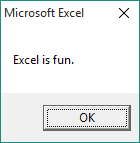String to Unicode and Back Results

### String to ASCII Decimal and Back

``````Sub StrConvDecimaltoASCII()
Dim str1 As String
Dim x() As Byte

str1 = "Excel is fun."
'store as Decimal Byte Array
x = StrConv(str1, vbFromUnicode)
MsgBox (StrConv(x, vbUnicode))
End Sub``````String to Decimal and Back Results

In case you’re a skeptic, here’s a screenshot showing the x decimal character array in the Watch Window.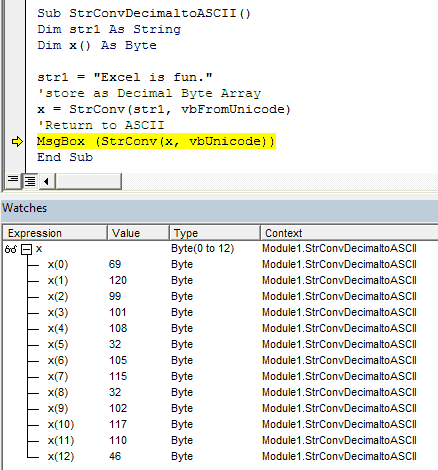VBA Character Array

Now that you believe me, go out and use these examples to store your strings in decimal or unicode byte arrays, manipulate them however you want in your VBA code, and return them back to ASCII characters when you’re ready.

## Final Thoughts

This was a big lesson. StrConv is a powerful function and is worthy of a big lesson. You’ve learned how to use the VBA StrConv function to convert strings to upper, lower and proper case. You’ve also learned how to use the same function to create byte arrays.

The StrConv function is great for making your strings pretty, but here’s a tip. You don’t have to convert your strings if all you want to do is to compare them and check for case insensitive matches. The StrComp function should be used for that.

That’s all for this tutorial. When you’re ready to take your VBA to the next level, subscribe using the form below.